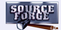Purchase Copyright © 2002 Paul Sheer. Click here for copying permissions. HomeNext: 24. Source and Binary Up: rute Previous: 22. Trivial Introduction to   Contents

Subsections

# 23. Shared Libraries

This chapter follows directly from our construction of static .a libraries in Chapter 22. It discusses creation and installation of Dynamically Linked Libraries (DLLs). Here I show you both so that you have a good technical overview of how DLLs work on UNIX. You can then promptly forget everything except ldconfig and LD_LIBRARY_PATH discussed below.

The .a library file is good for creating functions that many programs can include. This practice is called code reuse. But note how the .a file is linked into (included) in the executable mytest in Chapter 22. mytest is enlarged by the size of libsimple_math.a. When hundreds of programs use the same .a file, that code is effectively duplicated all over the file system. Such inefficiency was deemed unacceptable long before LINUX, so library files were invented that only link with the program when it runs--a process known as dynamic linking. Instead of .a files, similar .so ( shared object) files live in /lib/ and /usr/lib/ and are automatically linked to a program when it runs.

# 23.1 Creating DLL .so Files

Creating a DLL requires several changes to the Makefile on page:

 ` ` ` ` ` ` ` ` `5`` ` ` ` ` ` ` ` ` ` `10`` ` ` ` ` ` ` ` ` ` `15`` ` ` ` ` ` ` ` ` ` `20`` ` ` ` ` ` `OBJS      = simple_math_sqrt.o simple_math_pow.o` `LIBNAME   = simple_math` `SONAME    = libsimple_math.so.1.0.0` `SOVERSION = libsimple_math.so.1.0` `CFLAGS    = -Wall` ` ` `all:    lib\$(LIBNAME).so mytest` ` ` `mytest: lib\$(LIBNAME).so mytest.o` `        gcc \$(CFLAGS) -o [email protected] mytest.o -L. -l\${LIBNAME}` ` ` `lib\$(LIBNAME).so: \$(OBJS)` `        gcc -shared \$(CFLAGS) \$(OBJS) -lc -Wl,-soname -Wl,\$(SOVERSION) \` `                         -o \$(SONAME) && \` `        ln -sf \$(SONAME) \$(SOVERSION) && \` `        ln -sf \$(SONAME) lib\$(LIBNAME).so` ` ` `.c.o:` `        gcc -fPIC -DPIC \$(CFLAGS) -c -o \$*.o \$<` ` ` `clean:` `        rm -f *.o *.a *.so mytest`

The -shared option to gcc builds our shared library. The -W options are linker options that set the version number of the library that linking programs will load at runtime. The -fPIC -DPIC means to generate position-independent code, that is, code suitable for dynamic linking.

After running make we have

 ` ` ` ` ` ` ` ` `lrwxrwxrwx    1 root     root           23 Sep 17 22:02 libsimple_math.so -> libsimple_math.so.1.0.0` `lrwxrwxrwx    1 root     root           23 Sep 17 22:02 libsimple_math.so.1.0 -> libsimple_math.so.1.0.0` `-rwxr-xr-x    1 root     root         6046 Sep 17 22:02 libsimple_math.so.1.0.0` `-rwxr-xr-x    1 root     root        13677 Sep 17 22:02 mytest`

# 23.2 DLL Versioning

You may observe that our three .so files are similar to the many files in /lib/ and /usr/lib/. This complicated system of linking and symlinking is part of the process of library versioning. Although generating a DLL is out of the scope of most system admin tasks, library versioning is important to understand.

DLLs have a problem. Consider a DLL that is outdated or buggy: simply overwriting the DLL file with an updated file will affect all the applications that use it. If these applications rely on certain behavior of the DLL code, then they will probably crash with the fresh DLL. UNIX has elegantly solved this problem by allowing multiple versions of DLLs to be present simultaneously. The programs themselves have their required version number built into them. Try

 ` ` `ldd mytest`

which will show the DLL files that mytest is scheduled to link with:

 ` ` ` ` ` ` `libsimple_math.so.1.0 => ./libsimple_math.so.1.0 (0x40018000)` `libc.so.6 => /lib/libc.so.6 (0x40022000)` `/lib/ld-linux.so.2 => /lib/ld-linux.so.2 (0x40000000)`

At the moment, we are interested in libsimple_math.so.1.0. Note how it matches the SOVERSION variable in the Makefile. Note also how we have chosen our symlinks. We are effectively allowing mytest to link with any future libsimple_math.so.1.0.? (were our simple_math library to be upgraded to a new version) purely because of the way we have chosen our symlinks. However, it will not link with any library libsimple_math.so.1.1.?, for example. As developers of libsimple_math, we are deciding that libraries of a different minor [For this example we are considering libraries to be named libname .so.major .minor .patch]version number will be incompatible, whereas libraries of a different patch level will not be incompatible.

We could also change SOVERSION to libsimple_math.so.1. This would effectively be saying that future libraries of different minor version numbers are compatible; only a change in the major version number would dictate incompatibility.

# 23.3 Installing DLL .so Files

If you run ./mytest, you will be greeted with an error while loading shared libraries message. The reason is that the dynamic linker does not search the current directory for .so files. To run your program, you will have to install your library:

 ` ` ` ` ` ` `mkdir -p /usr/local/lib` `install -m 0755 libsimple_math.so libsimple_math.so.1.0 \` `                            libsimple_math.so.1.0.0 /usr/local/lib`

Then, edit the /etc/ld.so.conf file and add a line

 ` ` `/usr/local/lib`

 ` ` `ldconfig`

 ` ` ` ` `export LD_LIBRARY_PATH="\$LD_LIBRARY_PATH:/usr/local/lib"` `./mytest`